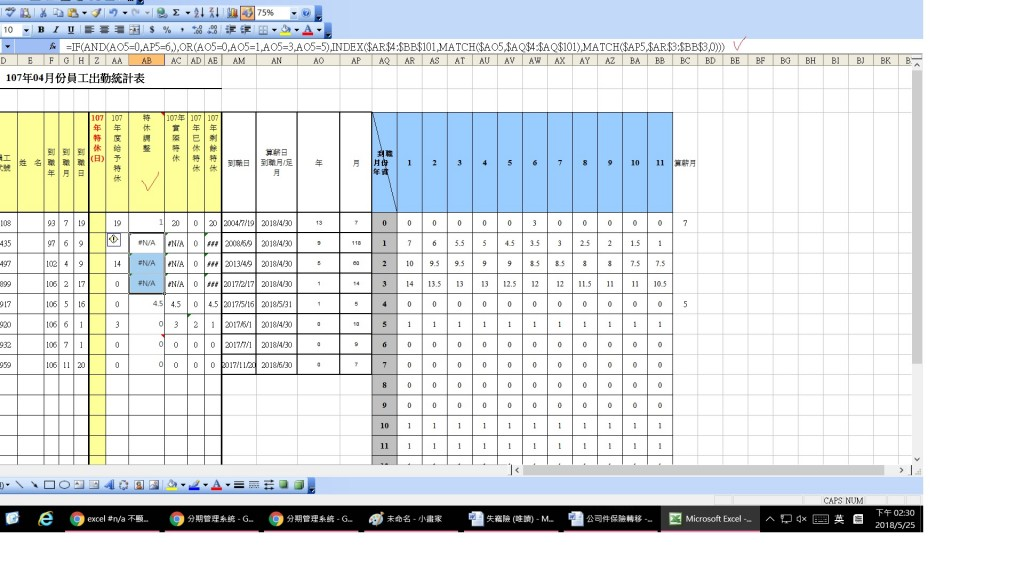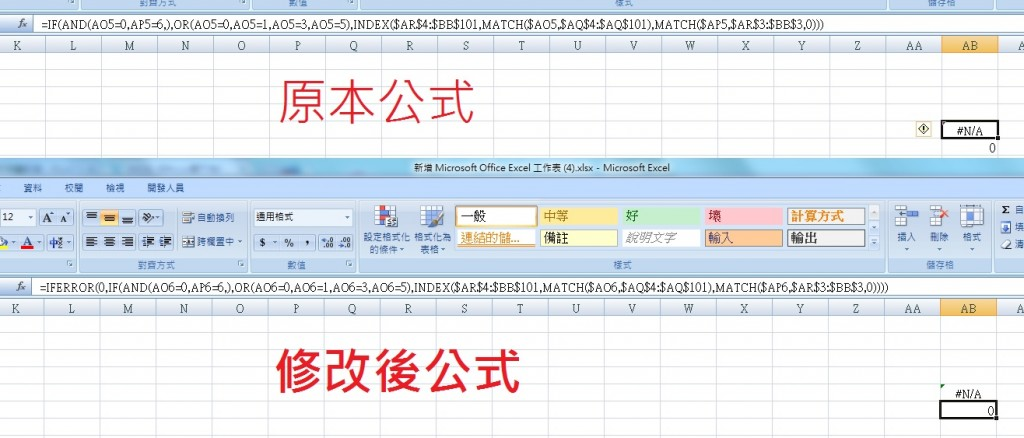#0

## EXCEL 把#n/a 顯示為0

EXCEL 把#n/a 顯示為0
AB 欄的公式 : =IF(AND(AO5=0,AP5=6,),OR(AO5=0,AO5=1,AO5=3,AO5=5),INDEX(\$AR\$4:\$BB\$101,MATCH(\$AO5,\$AQ\$4:\$AQ\$101),MATCH(\$AP5,\$AR\$3:\$BB\$3,0)))1

# 方法一、`IFNA()`函數

``````=IFNA(IF(AND(AO5=0,AP5=6,),OR(AO5=0,AO5=1,AO5=3,AO5=5),INDEX(\$AR\$4:\$BB\$101,MATCH(\$AO5,\$AQ\$4:\$AQ\$101),MATCH(\$AP5,\$AR\$3:\$BB\$3,0))),0)
``````

# 方法二、組合`IF()`與`ISNA()`函數

`IF(ISNA(express), 0, express))`

``````=IF(ISNA(IF(AND(AO5=0,AP5=6,),OR(AO5=0,AO5=1,AO5=3,AO5=5),INDEX(\$AR\$4:\$BB\$101,MATCH(\$AO5,\$AQ\$4:\$AQ\$101),MATCH(\$AP5,\$AR\$3:\$BB\$3,0)))), 0, IF(AND(AO5=0,AP5=6,),OR(AO5=0,AO5=1,AO5=3,AO5=5),INDEX(\$AR\$4:\$BB\$101,MATCH(\$AO5,\$AQ\$4:\$AQ\$101),MATCH(\$AP5,\$AR\$3:\$BB\$3,0))))
``````

# 題外話

`ISNA()`只會檢查是否為`#N/A`，如果你想要將其他的錯誤類型(例如：`NUM!``REF!`等)也轉成`0`的話，可以把`ISNA()`改為`ISERROR()`

swhung iT邦新手 5 級 ‧ 2018-05-25 15:24:44 檢舉

swhung iT邦新手 5 級 ‧ 2018-05-25 16:00:06 檢舉

0

=IFERROR(0,原本公式)swhung iT邦新手 5 級 ‧ 2018-05-25 15:44:14 檢舉